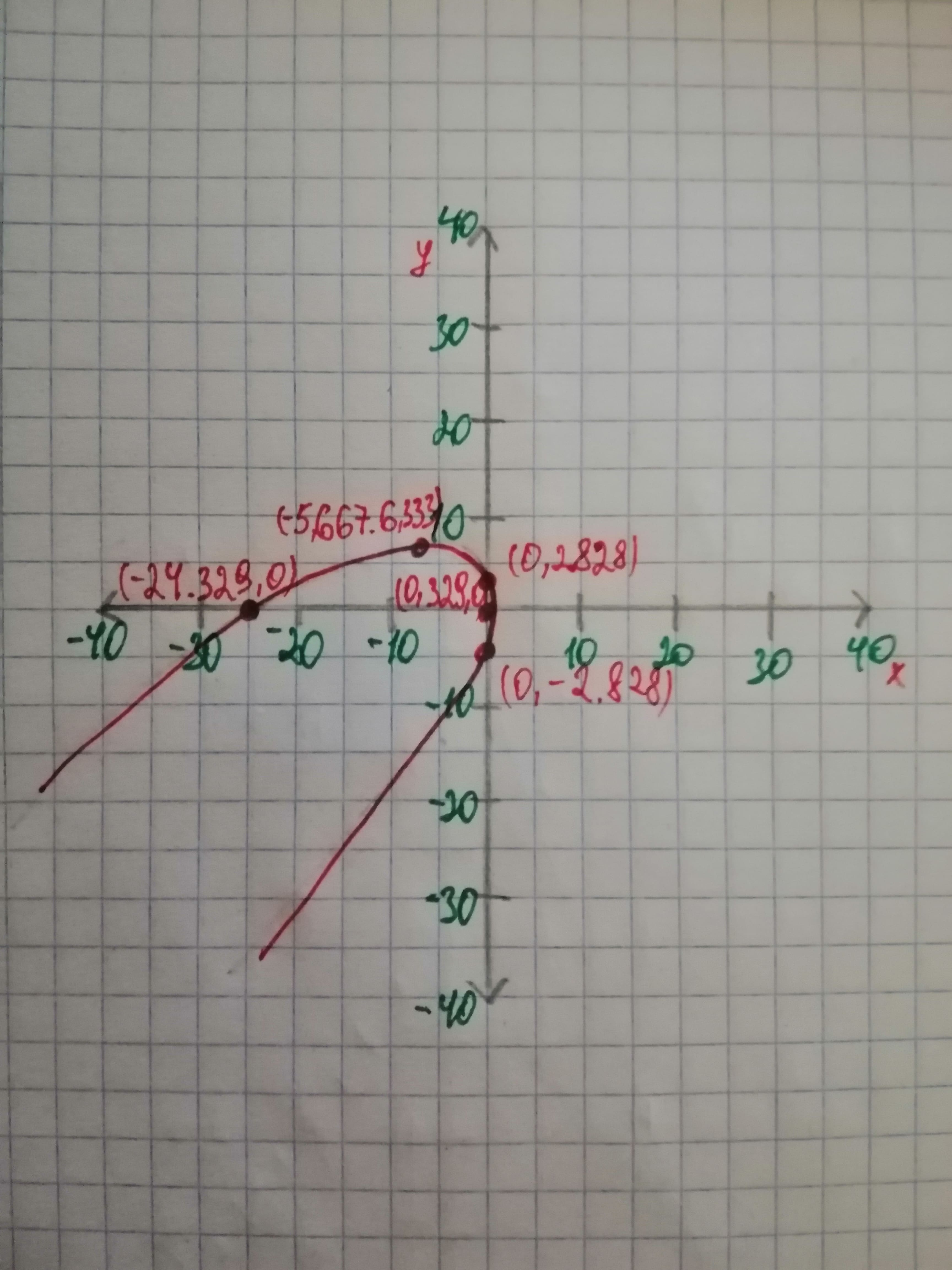Dillard

2021-01-05

The type of the conic section using the Discriminant Test and plot the curve using a computer algebra system.diskusje5

Concept Used: The general equation of conic has the discriminant $\mathrm{\Delta }$ and its given as The general equation of conic can also be written in the following way If $\mathrm{\Delta }\ne q0,$ then the equation is nondegenerate and under this case it represents a hyperbola and a rectangular hyperbola if
it represents a parabola. or ellipse $A\ne qC$ Calculations: The given equation is: and the equation is nondegenerate, hence $\mathrm{\Delta }\ne q0$. Now let's find the value of . From the given equation we have so on substitution we get Hence the given equation represents a Parabola. The plot of the given curve is as below:Conclusion: The given equation represents a Parabola and the plot is shown above.

Do you have a similar question?